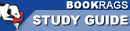Study Guide

# Journey Through Genius: The Great Theorems of Mathematics - A Sampler of Euler's Number Theory Summary & Analysis

This Study Guide consists of approximately 26 pages of chapter summaries, quotes, character analysis, themes, and more - everything you need to sharpen your knowledge of Journey Through Genius.
 This section contains 310 words (approx. 1 page at 400 words per page) View a FREE sample## A Sampler of Euler's Number Theory Summary and Analysis

Euler is also remarkable for his contributions to number theory, Dunham writes, and in this chapter he examines some of Euler's theorems concerning prime numbers and the earlier work of Fermat.

Fermat had made several observations about prime numbers which he claimed to have proven, but for which no proof is known at the time of Euler. Euler is able to prove some of Fermat's theorems, including the one called the "little Fermat theorem."

Euler also turns his attention to another of Fermat's claims, which is that 2²ⁿ + 1 is always a prime number. This is true for the first 4 values of n, but Euler discovers that the number produced by the formula where n=5, which is 4,294,967,297, is not prime. He is able to factor it, something no mathematician has been able to...

(read more from the A Sampler of Euler's Number Theory Summary)

 This section contains 310 words (approx. 1 page at 400 words per page) View a FREE sample